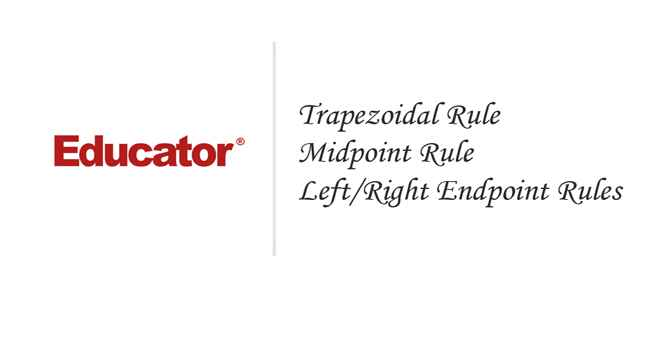Professor Murray

Trapezoidal Rule, Midpoint Rule, Left/Right Endpoint Rule

Slide Duration:

Integration by Parts

24m 52s

Intro
0:00
Important Equation
0:07
Where It Comes From (Product Rule)
0:20
Why Use It?
0:35
Lecture Example 1
1:24
Lecture Example 2
3:30
Shortcut: Tabular Integration
7:34
Example
7:52
Lecture Example 3
10:00
Mnemonic: LIATE
14:44
Ln, Inverse, Algebra, Trigonometry, e
15:38
-1
-2
Integration of Trigonometric Functions

25m 30s

Intro
0:00
Important Equation
0:07
Powers (Odd and Even)
0:19
What To Do
1:03
Lecture Example 1
1:37
Lecture Example 2
3:12
Half-Angle Formulas
6:16
Both Powers Even
6:31
Lecture Example 3
7:06
Lecture Example 4
10:59
-1
-2
Trigonometric Substitutions

30m 9s

Intro
0:00
Important Equations
0:06
How They Work
0:35
Example
1:45
Remember: du and dx
2:50
Lecture Example 1
3:43
Lecture Example 2
10:01
Lecture Example 3
12:04
-1
-2
Partial Fractions

41m 22s

Intro
0:00
Overview
0:07
Why Use It?
0:18
Lecture Example 1
1:21
Lecture Example 2
6:52
Lecture Example 3
13:28
-1
-2
Integration Tables

20m

Intro
0:00
Using Tables
0:09
Match Exactly
0:32
Lecture Example 1
1:16
Lecture Example 2
5:28
Lecture Example 3
8:51
-1
-2
Trapezoidal Rule, Midpoint Rule, Left/Right Endpoint Rule

22m 36s

Intro
0:00
Trapezoidal Rule
0:13
Graphical Representation
0:20
How They Work
1:08
Formula
1:47
Why a Trapezoid?
2:53
Lecture Example 1
5:10
Midpoint Rule
8:23
Why Midpoints?
8:56
Formula
9:37
Lecture Example 2
11:22
Left/Right Endpoint Rule
13:54
Left Endpoint
14:08
Right Endpoint
14:39
Lecture Example 3
15:32
-1
-2
Simpson's Rule

21m 8s

Intro
0:00
Important Equation
0:03
Estimating Area
0:28
Difference from Previous Methods
0:50
General Principle
1:09
Lecture Example 1
3:49
Lecture Example 2
6:32
Lecture Example 3
9:07
-1
-2
Improper Integration

44m 18s

Intro
0:00
Horizontal and Vertical Asymptotes
0:04
Example: Horizontal
0:16
Formal Notation
0:37
Example: Vertical
1:58
Formal Notation
2:29
Lecture Example 1
5:01
Lecture Example 2
7:41
Lecture Example 3
11:32
Lecture Example 4
15:49
Formulas to Remember
18:26
Improper Integrals
18:36
Lecture Example 5
21:34
Lecture Example 6 (Hidden Discontinuities)
26:51
-1
-2
Section 2: Applications of Integrals, part 2
Arclength

23m 20s

Intro
0:00
Important Equation
0:04
Why It Works
0:49
Common Mistake
1:21
Lecture Example 1
2:14
Lecture Example 2
6:26
Lecture Example 3
10:49
-1
-2
Surface Area of Revolution

28m 53s

Intro
0:00
Important Equation
0:05
Surface Area
0:38
Relation to Arclength
1:11
Lecture Example 1
1:46
Lecture Example 2
4:29
Lecture Example 3
9:34
-1
-2
Hydrostatic Pressure

24m 37s

Intro
0:00
Important Equation
0:09
Main Idea
0:12
Different Forces
0:45
Weight Density Constant
1:10
Variables (Depth and Width)
2:21
Lecture Example 1
3:28
-1
-2
Center of Mass

25m 39s

Intro
0:00
Important Equation
0:07
Main Idea
0:25
Centroid
1:00
Area
1:28
Lecture Example 1
1:44
Lecture Example 2
6:13
Lecture Example 3
10:04
-1
-2
Section 3: Parametric Functions
Parametric Curves

22m 26s

Intro
0:00
Important Equations
0:05
Slope of Tangent Line
0:30
Arc length
1:03
Lecture Example 1
1:40
Lecture Example 2
4:23
Lecture Example 3
8:38
-1
-2
Polar Coordinates

30m 59s

Intro
0:00
Important Equations
0:05
Polar Coordinates in Calculus
0:42
Area
0:58
Arc length
1:41
Lecture Example 1
2:14
Lecture Example 2
4:12
Lecture Example 3
10:06
-1
-2
Section 4: Sequences and Series
Sequences

31m 13s

Intro
0:00
Definition and Theorem
0:05
Monotonically Increasing
0:25
Monotonically Decreasing
0:40
Monotonic
0:48
Bounded
1:00
Theorem
1:11
Lecture Example 1
1:31
Lecture Example 2
11:06
Lecture Example 3
14:03
-1
-2
Series

31m 46s

Intro
0:00
Important Definitions
0:05
Sigma Notation
0:13
Sequence of Partial Sums
0:30
Converging to a Limit
1:49
Diverging to Infinite
2:20
Geometric Series
2:40
Common Ratio
2:47
Sum of a Geometric Series
3:09
Test for Divergence
5:11
Not for Convergence
6:06
Lecture Example 1
8:32
Lecture Example 2
10:25
Lecture Example 3
16:26
-1
-2
Integral Test

23m 26s

Intro
0:00
Important Theorem and Definition
0:05
Three Conditions
0:25
Converging and Diverging
0:51
P-Series
1:11
Lecture Example 1
2:19
Lecture Example 2
5:08
Lecture Example 3
6:38
-1
-2
Comparison Test

22m 44s

Intro
0:00
Important Tests
0:01
Comparison Test
0:22
Limit Comparison Test
1:05
Lecture Example 1
1:44
Lecture Example 2
3:52
Lecture Example 3
6:01
Lecture Example 4
10:04
-1
-2
Alternating Series

25m 26s

Intro
0:00
Main Theorems
0:05
Alternation Series Test (Leibniz)
0:11
How It Works
0:26
Two Conditions
0:46
Never Use for Divergence
1:12
Estimates of Sums
1:50
Lecture Example 1
3:19
Lecture Example 2
4:46
Lecture Example 3
6:28
-1
-2
Ratio Test and Root Test

33m 27s

Intro
0:00
Theorems and Definitions
0:06
Two Common Questions
0:17
Absolutely Convergent
0:45
Conditionally Convergent
1:18
Divergent
1:51
Missing Case
2:02
Ratio Test
3:07
Root Test
4:45
Lecture Example 1
5:46
Lecture Example 2
9:23
Lecture Example 3
13:13
-1
-2
Power Series

38m 36s

Intro
0:00
Main Definitions and Pattern
0:07
What Is The Point
0:22
0:45
Interval of Convergence
2:42
Lecture Example 1
3:24
Lecture Example 2
10:55
Lecture Example 3
14:44
-1
-2
Section 5: Taylor and Maclaurin Series
Taylor Series and Maclaurin Series

30m 18s

Intro
0:00
Taylor and Maclaurin Series
0:08
Taylor Series
0:12
Maclaurin Series
0:59
Taylor Polynomial
1:20
Lecture Example 1
2:35
Lecture Example 2
6:51
Lecture Example 3
11:38
Lecture Example 4
17:29
-1
-2
Taylor Polynomial Applications

50m 50s

Intro
0:00
Main Formulas
0:06
Alternating Series Error Bound
0:28
Taylor's Remainder Theorem
1:18
Lecture Example 1
3:09
Lecture Example 2
9:08
Lecture Example 3
17:35
-1
-2
Bookmark & Share Embed

## Copy & Paste this embed code into your website’s HTML

Please ensure that your website editor is in text mode when you paste the code.
(In Wordpress, the mode button is on the top right corner.)
×
• - Allow users to view the embedded video in full-size.
Since this lesson is not free, only the preview will appear on your website.

• ## Related Books1 answer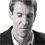Last reply by: Dr. William MurrayFri Jun 27, 2014 4:59 PMPost by Jorge Sardinas on June 24, 2014Dear Dr.Murraythank for all the wonderful lectures and i just wanted to let you know that you are an amazing teacherP.S. I also love your trigonometry lessons.1 answerLast reply by: Dr. William MurrayTue Jan 7, 2014 11:29 AMPost by Edmund Mercado on December 20, 2013Dr. Murray:Isn't the calculator required to be in radian mode for these solutions?1 answerLast reply by: Dr. William MurrayMon Nov 12, 2012 4:47 PMPost by Justin Malaer on November 10, 2012Do you guys even answer questions?1 answerLast reply by: Dr. William MurrayTue May 14, 2013 9:55 AMPost by Jess Wood on October 15, 2011In the last example of the left endpoint rule, there is a 1/2 that is multiplied throughout the added height of the left endpoints. Is that 1/2 suppose to be 1/4? If not how did the 1/2 come about?

### Trapezoidal Rule, Midpoint Rule, Left/Right Endpoint Rule

Main formula: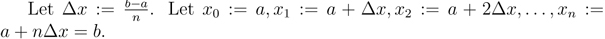Trapezoidal Rule: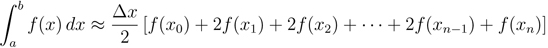Midpoint Rule: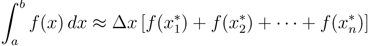where,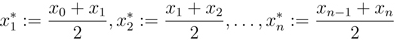Left/Right Endpoint Rules: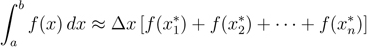where for the Left Endpoint Rule,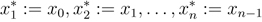For the Right Endpoint Rule,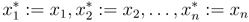Hints and tips:

• These formulas may seem long and complicated. Instead of memorizing them, remember the geometry on which they’re based. If you can draw the pictures of the trapezoids and rectangles, you can probably reconstruct the formulas quickly.

• The Midpoint Rule is more accurate than the Trapezoid Rule, even though it is simpler and requires fewer function evaluations.

• By graphing the curve that you’re estimating the area under, you can often tell whether the estimates from the various formulas will be higher or lower than the true area.

• Sometimes you will not have a graph of the function or an explicit formula. Instead, you will use one of these Rules to estimate integrals based on data from charts.

### Trapezoidal Rule, Midpoint Rule, Left/Right Endpoint Rule

Lecture Slides are screen-captured images of important points in the lecture. Students can download and print out these lecture slide images to do practice problems as well as take notes while watching the lecture.

• Intro 0:00
• Trapezoidal Rule 0:13
• Graphical Representation
• How They Work
• Formula
• Why a Trapezoid?
• Lecture Example 1 5:10
• Midpoint Rule 8:23
• Why Midpoints?
• Formula
• Lecture Example 2 11:22
• Left/Right Endpoint Rule 13:54
• Left Endpoint
• Right Endpoint
• Lecture Example 3 15:32

### Transcription: Trapezoidal Rule, Midpoint Rule, Left/Right Endpoint Rule

We are going to do a couple more examples here.0000

I want to get some more practice with the midpoint rule.0003

We are going to estimate the integral from 1 to 2 of x × ln(x).0006

Now, here b = 2, a = 1.0011

So, δx, which is b-a/n, is 2-1/4, so that is 1/4.0020

The midpoint rule says the integral is approximately equal to 1/4 × f(the midpoint of these 4 intervals).0030

So if we take the interval from 1 to 2 and split it into 4 pieces, that is 1 and 1/4, 1 and 1/2, 1 and 3/4.0042

Now we want the midpoints of those 4 integrals.0050

The midpoint of the first one is 1 and 1/8.0056

The midpoint of the second one between 1 and 1/4 and 1 and 1/2 is 1 and 3/8.0064

The midpoint of the third one is 1 and 5/8.0072

The last one is 1 and 7/8.0076

That is 1/4.0081

Now the function here, we get from the integral.0084

That is x ln(x), so that is 1 and 1/8, we want to plug that in there.0088

1 and 1/8 is 1.125 × ln(1.125).0095

We plug each one of these values in there and I will not write them all down, but the last one here is 1 and 7/8.0102

Plug that in, and we get 1.875 × ln(1.875).0110

That is an expression now that you could plug into your calculator.0123

You just take these numbers and these expressions and plug them all into your calculator.0128

What you come up with is .634493.0135

So, that tells us that our integral is approximately equal to .634493.0143

Notice there that we never actually solve the integral as we would have using some of our earlier techniques in Calculus 2.0154

We just picked different points and plugged them into the function and got an approximation of the area.0160

So the next example I would like to do is using the right endpoint rule.0000

We are going to find the integral of sin(x) from 1 to 2.0007

It says to use n = 4, so we will split that into 4 pieces.0013

δx is b-a/n, so that is 2/1/4, which is 1/4.0019

Then, the right endpoint rule says you take the width of these rectangles, which is 1/4, that is the δx...0028

... times, now you plug in for the height, you plug in the right endpoint.0037

So we are not going to look at the left endpoint of the first interval.0040

We are going to look at the right endpoint of those four intervals.0047

So we look at f(1 and 1/4) + f(1 and 1/2) + f(1 and 3/4) +f(2).0050

f(2) represents the right endpoint of the last interval there.0065

Now the function f here is sin(x), so we will be doing sin(1.25) + sin(1.5) + sin(1.75) + sin(2).0073

Again, this is something you can plug into your calculator.0093

When I plugged it into my calculator and simplified it down,0100

I got .959941 as an answer.0103

So, that is the approximate area under sin(x) using the right endpoint rule without actually doing any actual integration.0112

That is the end of the lecture on approximate integration.0126

We covered the trapezoid rule, the midpoint rule, and the left and right endpoint rules.0128

Those were all brought to you by educator.com.0138

This is educator.com, and today we are going to discuss three methods of integration approximation, the trapezoidal rule, the midpoint rule, and the left and right end-point rules.0000

The idea here is that you are trying to approximate the area under a curve0014

The function here is f(x) and we are trying to approximate that from x=a to x=b and we are trying to find that area.0027

What you have done so far in your calculus class, is you just take the integral of f and then you plug in the endpoints.0044

The point is that there are a lot of functions that you will not be able to take the integral of directly.0050

So, what we are going to try to do is find approximation techniques that do not rely on us being able to take the integral.0055

The idea for all of these techniques is that you start out by dividing the region between a to b into n equal partitions.0062

Then we are going to look at the area on each one of those.0082

That is the first part of the formula here.0086

Each one of these partitions is δx y, and δx comes from b - a, that is the total width, divided by n because there are n of these segments.0093

Now on the trapezoidal rule, what we are going to do is label each one of these points on the x axis.0108

This is x0, x1, x2 all the way up to xn is b.0115

That is where the next part of this formula comes from.0124

x0 is a, x1 is a + δX because it is a and then you go over δx, x2 is a + 2 δX all the way up to xn is a + n δx.0127

But, of course a + n δx is a + b - a/n.0147

So, that is a + b -a which is b, so xn is the same as b0156

We have labelled these points on the x axis, and what we are trying to do is approximate the area.0162

What we do to approximate the area is we are going to use several different rules.0172

The first one is called the trapezoidal rule.0179

The trapezoidal rule means that you draw little trapezoids on each of these segments, and then you find the area of these trapezoids.0183

The area of a trapezoid is = 1/2(base1 × base2 × height).0200

That area of the trapezoid is reflected in this formula right here.0218

The 1/2 gives you that 2 right there, the height of a trapezoid, that is the height, and that is the width of one of those trapezoids, and that is δX0225

Then you have base1 + base2 is, I am going to show this in red, base1 + base2, that is for the first trapezoid.0243

For the next trapezoid, base1 + base2, and so on, up to the last trapezoid, base1 + base2.0260

What you are doing is you are plugging each of these x0, x1, x2 into f to get these heights0279

But you only have 1 of the end one and 2 of each of the middle ones.0287

That is why you get 1 here, and two of each of the middle ones, and one of the end one.0297

So, that is where the formula for the trapezoidal rule comes from.0305

Let us try it out on an example.0308

Example 1 is we are going to try to estimate the integral from 1 to 2 of sin(x) dX.0313

Here is 1, and here is 2, and we are going to try to estimate that using n = 4.0322

That means we are going to divide the region from 1 to 2 into 4 pieces.0330

Using the formula for the trapezoid rule, we have δx/2.0336

Well δx is (b - a)/n, so that is 1/4.0341

δx/2 is 1/8, so we are going to have 1/8 times f(x0) + 2f(x1) + 2f(x2) + 2f(x3) + only 1 of f(x4).0352

These x0's are the division points in between 1 and 2, so this x0 is 1, so that is sin(1) + 2, now that is 1, x1 × δx is 1/4.0385

x2 can go over another unit of δx, so that is 1 and 1/2, 1 and 3/4 and finally, sin(2).0410

We are going to take all of that, and multiply it by 1/8.0430

At this point it is simply a matter of plugging all of these values into a calculator.0433

I have a TI calculator here, and I am going to plug in 1/8 sin(1) is 0.01745 + 2sin(1.25), which is 0.0281, and so on.0437

You can plug the rest of the values into a calculator.0468

What you get at the end simplifies down to 0.951462.0476

So, we say that the integral from 1 to 2 of sin(x) dX is approximately = 0.9514620486

Next rule we are going to learn is the midpoint rule.0503

It is the same idea, where you have a function that you want to integrate from a to b, and you break the region up into partitions.0508

So, you have x0 = a, xn = b and a bunch of partitions in between, each partition is δx y.0522

Except, in each partition, instead of building trapezoids, we are going to build rectangles.0533

We are going to build rectangles on the height of the middle of the partition.0542

Here, we are going to look at the middle of the partition,0548

We see how tall the function is at the middle of the partition and we build a rectangle that is that height.0555

We do that on every rectangle.0564

The formula we get in total is δx, that is the width of the rectangles, times the height of these rectangles,0575

I have labelled the midpoints of those rectangles x1* and x2* and xn*.0596

Those represent these midpoints, so that is x1*, there is x2*, and so on.0616

Those are the midpoints so x1* is just x0 + x1/2, x2* is just x2 + x2/2,0622

And so on and those just represent the midpoints of each of these intervals.0632

We plug those midpoints in to find the heights of the rectangles and estimate the area.0640

What we are going to do for the last rule today, is we are going to use instead of the midpoints, we will use the left endpoints of each rectangle.0650

Instead of having to find the midpoints, the x1× and the x2× and so on will be the left endpoint of each interval.0663

We will use those to get the heights.0677

We will see those in the second.0680

First we will do an example with the midpoint rule using the same integral as before.0682

Sin(x), there is 1, there is 2, again we are using n = 4 so we will break it up into 4 partitions.0686

Except we are going to use a slightly different formula to solve it.0698

Again, δx = 1/4, because that is the width of each of these rectangles, but now we are going to look at the midpoints of those 4 rectangles to find the heights.0700

The midpoints are, well, this is 1 right here, that is 1 and 1/4, the midpoint there is 1 and 1/8.0715

The next midpoint is halfway between 1 and 1/4 and 1 and 1/2 and that is 1 and 3/8.0731

The formula that we get is δX(f(1 + 1/8) + f(1 + 3/8) + f(1 + 5/8) + f(1 + 7/8) and that is just 1/4.0740

Now the function here is sin(x) so we will be doing sin(1 + 1/8), that is 1.125, + sin(1 + 3/8), that is 1.375, sin(1 + 5/8) is 1.625, and sin(1 + 7/8) is 1.875.0771

Now it is just an expression that we can plug into our calculator.0800

I worked this out ahead of time, I got 0.9589440805

That is our best approximation for the integral using the midpoint rule.0827

The next formula we want to learn is the right and left endpoint rule.0835

We will talk about the left endpoint rule first.0840

It is pretty much the same as the midpoint rule.0845

Again, you are drawing these rectangles except instead of using the midpoint to find the height of the rectangle, you are using the left endpoint.0849

So that means you start out with the exact same formula,0862

Except that the star points that you choose to plug in to find the heights are just the left endpoints, x0, x1, up to xn-1.0865

You do not go up to xn.0876

For the right endpoint rule it is the same formula except you use the right endpoints.0879

The right endpoint would be x1, x2, up to xn.0885

You do not have x0 anymore because that is the first endpoint of the left formula.0890

Let me draw these in different colors here.0896

The left endpoints give you x0, x1, up to xn-1, so that is the left endpoint rule.0902

The right endpoint, I will do that one in blue, is x1, x2, up to xn-1, and xn.0912

You are using the right endpoints so that is the right endpoint rule.0928

We will do another example.0932

Again we are going to figure out the integral, or estimate the integral of sin(x) from 1 to 2.0934

We are going to use the left endpoint rule so that means the key points that we plug in for the heights are 1, 1 + 1/4, 1 + 1/2, and 1 + 3/4.0947

Again δx = 1/4 and our formula says δx × f(left endpoint).0964

So, f(1) + f(1 + 1/4) + f(1 + 1/2) + f(1 + 3/4).0975

We do not go to 2 because that was the right endpoint of the left interval.0990

The integral is approximately equal to that.1000

That is sin(1) + sin(1 + 1/4) + sin(1 + 1/2) + sin(1 + 3/4).1010

That is something that you can plug into your calculator, and when I did that ahead of time I got 0.942984.1022

That is our estimation of that integral using the left endpoint rule.1038

OR

### Start Learning Now

Our free lessons will get you started (Adobe Flash® required).